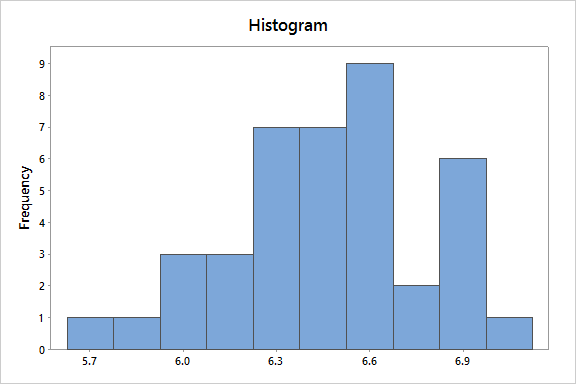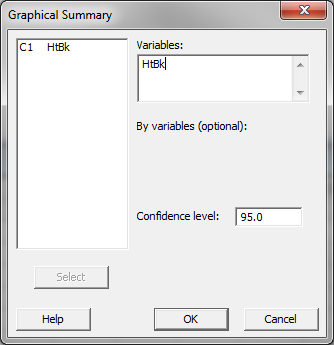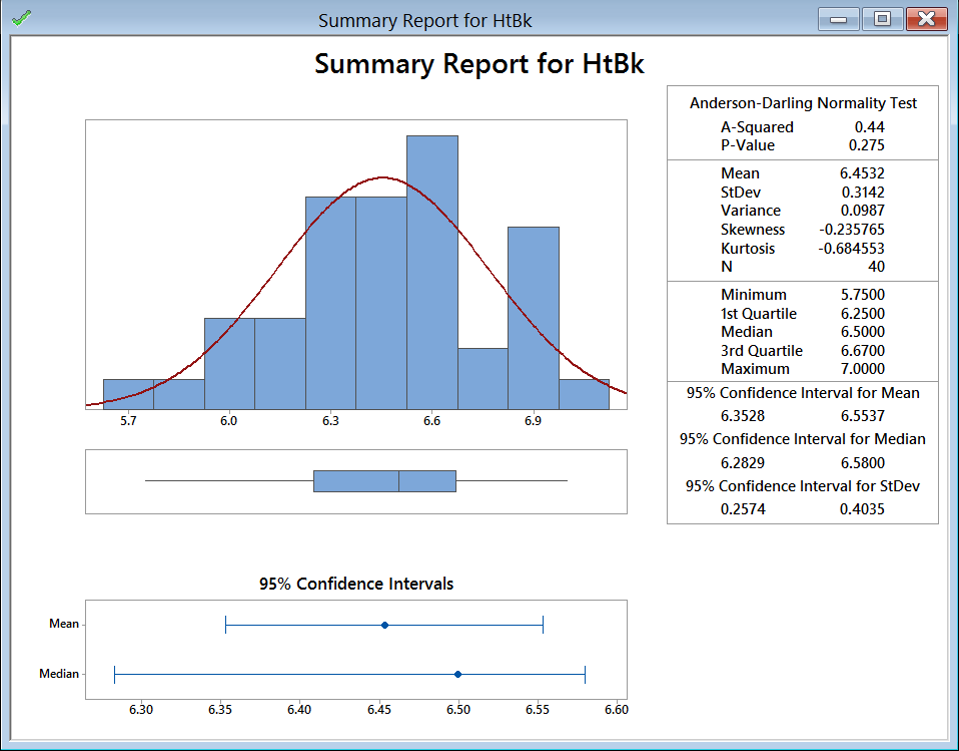# Histogram Rendering with Minitab

### What is a Histogram?

A histogram is a graphical tool to present the distribution of the data. The X axis represents the possible values of the variable and the Y axis represents the frequency of the value occurring. This graphical summary consists of adjacent rectangles erected over intervals with heights equal to the frequency density of the interval. The total area of all the rectangles is the number of data values.A histogram can also be normalized. In the case of normalization, the X axis still represents the possible values of the variable, but the Y axis represents the percentage of observations that fall into each interval on the X axis. The total area of all the rectangles in a normalized histogram is 1. When using these graphical representations, we have a better understanding of the shape, location, and spread of the data.

### How to Use Minitab to Generate a Histogram

Data File: “Histogram” tab in “Sample Data.xlsx”
Steps to render in Minitab:

1. Click Stat → Basic Statistics → Graphical Summary.
2. A new window named “Graphical Summary” pops up.
3. Select “HtBk” as the “Variables.”4. Click “OK.”
5. The histogram appears in the new window.Model summary: The output from the previous steps has generated a graphical summary report of the data set HtBk. Among the information provided is a histogram. The image shows the frequency of the data for the numerical categories ranging from 5.7 to approximately 6.9. You can see the shape of the data roughly follows the bell curve.